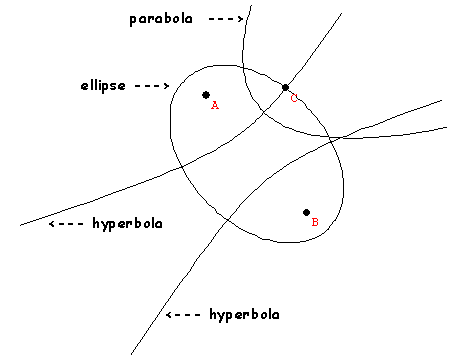Thursday,  April 14 (outline)

Projective Geometry- Calculus Beginnings
The conics.
Cones and Conic SectionsFAPP video.

Lines - Ratios, Rates, Proportions-Measurement.
• Average Speed and velocity
• Delta Notation
• More on Similar triangles
• Slopes of lines
Parabolas
• Line of symmetry
• Coordinate graphs of quadratics relation
• Finding line of symmetry from data, from equation
• Secant lines and slopes
• Locatring the vertex. and slopes of secant lines.
Coordinates and Functions.
• Functions rule relating variables
• function notation x -> f(x)
Section 10.0 - ON-Line Quiz
Tutorial on Functions (numerical & algebraic)
Tutorial on Functions (Visual/Graphical)

Static vs. Dynamic visualizations
Winplot?

Ch. 10.1 Some Historical Problems of Visualization:
• The parabola and squares.
• Ratio of Length of verticals :: ratio of squares on horizontals
• Visualizing Algebra, Motion and Change
• time vs. position
• time vs velocity
• Analytic geometry- Descartes and Fermat
• Section 10.1 - Quiz

10.2  Four Problems Connecting the visual to the Numerical
• Area of a region.
• Tangent line
• Motion and distance traveled by a falling object
• Motion and position (cannon balls)
• Section 10.2 - Quiz